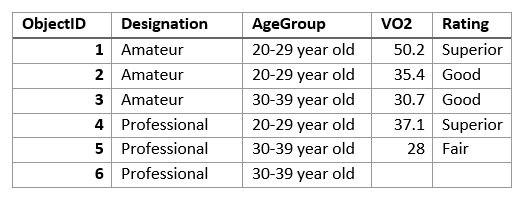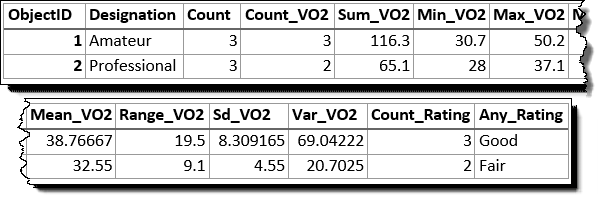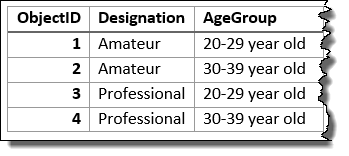# Summarize Attributes (GeoAnalytics Desktop)

## Summary

Calculates summary statistics for fields in a feature class.

## Usage

• Summarize Attributes is a tabular analysis tool, not a spatial analysis tool. Inputs can be a tabular layer or a layer with geometry (points, lines, or polygons).

• You can specify one or more fields to summarize by or summarize all features. When you summarize by fields, statistics are calculated for each unique combination of attribute values.

• The output table will consist of fields containing the result of the statistical operation.

• A field will be created for each specified statistic type using the following naming convention: sum_<field>, max_<field>, min_<field>, range_<field>, std_<field>, count_<field>, var_<field>, and any_<field> (where <field> is the name of the input field for which the statistic is computed). The statistics will be calculated on each group separately.

• You can apply this tool to spatial data, and you will get a tabular result. You can join your results to spatial data using Join Features.

• If time is enabled on the input, you can apply time stepping to your analysis. Each time step is analyzed independent of features outside the time step. To use time stepping, your input data must be time enabled and represent an instant in time. When time stepping is applied, output features will be time intervals represented by the START_DATETIME and END_DATETIME fields.

• The tables below illustrate the statistical calculations of a layer that is summarized using like values of fields. The VO2 field was used to calculate the numeric statistics (Count,Sum, Minimum, Maximum, Range, Mean, Standard Deviation, and Variance) for the layer. The Rating field was used to calculate the string statistics (Count and Any) for the layer.The input layer to be summarized is shown.

The table above was summarized on the Designation field, and the VO2 field was used to calculate the numeric statistics (Count,Sum, Minimum, Maximum, Range, Mean, Standard Deviation, and Variance) for the layer. The Rating field was used to calculate the string statistics (Count and Any) for the layer. This results is a table with two features, representing the distinct values of Designation.The input layer that has been summarized using the Designation field is shown.

The following table represents what the first few fields look like when the layer is summarized using the Designation and Age Group fields. Statistics are calculated using the same methods as the previous example.The input layer that has been summarized using the Designation and Age Group fields is shown.
• You can improve the performance of the Summarize Attributes tool by using the following tips:

• Set the extent environment so you only analyze data of interest.
• Use data that is local to where the analysis is being run.

• This geoprocessing tool is powered by Spark. Analysis is completed on your desktop machine using multiple cores in parallel. See Considerations for GeoAnalytics Desktop tools to learn more about running analysis.

• When running GeoAnalytics Desktop tools, the analysis is completed on your desktop machine. For optimal performance, data should be available on your desktop. If you are using a hosted feature layer, it is recommended that you use ArcGIS GeoAnalytics Server. If your data isn't local, it will take longer to run a tool. To use your ArcGIS GeoAnalytics Server to perform analysis, see GeoAnalytics Tools.

• Similar analysis can also be completed using the Summary Statistics tool in the Analysis toolbox.

## Parameters

 Label Explanation Data Type Input Layer The point, polyline, or polygon layer to be summarized. Table View Output Table A new table with the summarized attributes. Table Fields A field or fields used to summarize similar features. For example, if you choose a single field called PropertyType with the values of commercial and residential, all of the fields with the value residential fields will be summarized together, with summary statistics calculated, and all of the fields with the value commercial will be summarized together. This example will results in two rows in the output, one for commercial, and one for residential summary values. You can optionally select no fields and summarize all features in a single summary result. Field Summary Fields (Optional) The statistics that will be calculated on specified fields. Value Table Time step interval (Optional) A value that specifies the duration of the time step. This parameter is only available if the input points are time enabled and represent an instant in time. Time stepping can only be applied if time is enabled on the input. Time Unit Time step repeat (Optional) A value that specifies how often the time-step interval occurs. This parameter is only available if the input points are time enabled and represent an instant in time. Time Unit Time step reference (Optional) A date that specifies the reference time with which to align the time steps. The default is January 1, 1970, at 12:00 a.m. This parameter is only available if the input points are time enabled and represent an instant in time. Date

`arcpy.gapro.SummarizeAttributes(input_layer, out_table, fields, {summary_fields}, {time_step_interval}, {time_step_repeat}, {time_step_reference})`
 Name Explanation Data Type input_layer The point, polyline, or polygon layer to be summarized. Table View out_table A new table with the summarized attributes. Table fields[fields,...] A field or fields used to summarize similar features. For example, if you choose a single field called PropertyType with the values of commercial and residential, all of the fields with the value residential fields will be summarized together, with summary statistics calculated, and all of the fields with the value commercial will be summarized together. This example will results in two rows in the output, one for commercial, and one for residential summary values. You can optionally select no fields and summarize all features in a single summary result. Field summary_fields[summary_fields,...](Optional) The statistics that will be calculated on specified fields. COUNT—The number of nonnull values. It can be used on numeric fields or strings. The count of [null, 0, 2] is 2.SUM—The sum of numeric values in a field. The sum of [null, null, 3] is 3.MEAN—The mean of numeric values. The mean of [0,2, null] is 1.MIN—The minimum value of a numeric field. The minimum of [0, 2, null] is 0.MAX—The maximum value of a numeric field. The maximum value of [0, 2, null] is 2.STDDEV—The standard deviation of a numeric field. The standard deviation of  is null. The standard deviation of [null, 1,1,1] is null.VAR—The variance of a numeric field in a track. The variance of  is null. The variance of [null, 1,1,1] is null.RANGE—The range of a numeric field. This is calculated as the minimum value subtracted from the maximum value. The range of [0, null, 1] is 1. The range of [null, 4] is 0.ANY—A sample string from a field of type string. Value Table time_step_interval(Optional) A value that specifies the duration of the time step. This parameter is only available if the input points are time enabled and represent an instant in time. Time stepping can only be applied if time is enabled on the input. Time Unit time_step_repeat(Optional) A value that specifies how often the time-step interval occurs. This parameter is only available if the input points are time enabled and represent an instant in time. Time Unit time_step_reference(Optional) A date that specifies the reference time with which to align the time steps. The default is January 1, 1970, at 12:00 a.m. This parameter is only available if the input points are time enabled and represent an instant in time. Date

### Code sample

SummarizeAttributes example (stand-alone script)

The following stand-alone script demonstrates how to use the SummarizeAttributes tool.

``````# Name: Summarize Attributes.py
# Description: Summarize Crime Data by year and beat.

# Import system modules
import arcpy

arcpy.env.workspace = "C:/data/CityData.gdb"

# Set local variables
inFeatures = "ChicagoCrimes"
summaryFields = ["Year", "Beat"]
summaryStatistics = [["Arrest", "COUNT"], ["District", "COUNT"]]
out = 'SummarizeCrimes'

# Execute SummarizeAttributes
arcpy.gapro.SummarizeAttributes(inFeatures, out, summaryFields,
summaryStatistics)``````

• Basic: No
• Standard: No